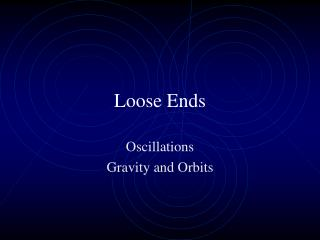Download PresentationLoose Ends

# Loose Ends

Download Presentation## Loose Ends

- - - - - - - - - - - - - - - - - - - - - - - - - - - E N D - - - - - - - - - - - - - - - - - - - - - - - - - - -
##### Presentation Transcript

1. Loose Ends Oscillations Gravity and Orbits

2. Oscillations • The connection between uniform circular motion and SHM • There is a close connection between circular motion and simple harmonic motion. Consider an object experiencing uniform circular motion, such as a mass sitting on the edge of a rotating turntable. This is two-dimensional motion, and the x and y position of the object at any time can be found by applying the following equations:

3. x = r cos θ y = r sin θ

4. Uniform Circular Motion • The motion is uniform circular motion, meaning that the angular velocity is constant, and the angular displacement is related to the angular velocity by the equation: θ = ωt • Plugging this in to the x and y positions makes it clear that these are the equations giving the coordinates of the object at any point in time, assuming the object was at the position x = r on the x-axis at time = 0: • x = rcos ωt and • y = rsin ωt

5. How does this relate to simple harmonic motion? An object experiencing simple harmonic motion is traveling in one dimension, and its one-dimensional motion is given by an x or y in the above equations.Where A is the amplitude. x = Acos ωt and y = Asin ωt

6. Period and Frequency • The amplitude is simply the maximum displacement of the object from the equilibrium position. • So, in other words, the same equation applies to the position of an object experiencing simple harmonic motion and one dimension of the position of an object experiencing uniform circular motion. Note that the in the SHM displacement equation is known as the angular frequency. It is related to the frequency (f) of the motion, and inversely related to the period (T):

7. Simple Harmonic Motion (SHM)

8. In simple harmonic motion, the velocity constantly changes, oscillating just as the displacement does. When the displacement is maximum, however, the velocity is zero; when the displacement is zero, the velocity is maximum. It turns out that the velocity is given by: v = -Aω sin ωt Where Aω is the maximum speed Velocity of an oscillating particle

9. a = -ω2x All of the equations above, for displacement, velocity, and acceleration as a function of time, apply to any system undergoing simple harmonic motion. What distinguishes one system from another is what determines the frequency of the motion. We'll look at that for two systems, a mass on a spring, and a pendulum Acceleration

10. The frequency of the motion for a mass on a spring • For SHM, the oscillation frequency depends on the restoring force. For a mass on a spring, where the restoring force is F = -kx, this gives: • F = md2x/dt2= -kx • If we integrate this equation we get x = Acos (ωt + θ) where ω = 2πf = √(k/m)

11. Physical Pendulum • Has an extended body • Torque = Iα • mgL sin θ = I(d2 θ/dt2) • Therefore ω = √(mgL/I) • Using this equation we can solve a problem.

12. Giraffe’s Leg • If a giraffe has a leg length of 1.8 m and a step length of 1 m, what would you estimate to be the period of its leg swing? • How fast would it then travel when walking?

13. Solution for the giraffe… • We can model a giraffe’s leg as a physical pendulum of length L pivoted about one end. I = 1/3 mL2. • T = 2π/ω = 2π√(I/mgL) • T = 2π √(mL2/3mgL) = 2π√(L/3g) = 1.6 sec • v = x/t = 1m/1.6 s = 0.6 m/s

14. Problems • A mass undergoes SHM with an amplitude of A. What fraction of the energy is kinetic energy when x = A/2? • A grandfather clock is to be designed so that on every half swing a small gear is moved one notch to indicate the passage of 1 s. What is the length required of the pendulum?

15. The total mechanical energy of the simple harmonic oscillator is constant (independent of time). However, the kinetic and potential energies are functions of time. • Torsion Pendulum-operation is associated with the twisting of a suspension wire. • Motion is called angular simple harmonic motion • Restoring torque τ = -κθ where kappa is a constant dependent on the suspension wire (length, diameter and material) • τ =Iα and α = d2θ/dt2 = τ/I = -(κ/I) θ

16. Comparing to linear quantities • ω2 = κ/I • The period of the Torsion Pendulum is • T = 2π/ω = 2π√(I/κ)

17. Example: Two springs are attached to a block of mass m and to fixed supports as shown in above. Show that the frequency of oscillation on the frictionless surface is given by . When spring 1 is extended by x, spring 2 is compressed by the same distance. The total force acting on the mass is the sum of the forces exerted by these two springs. Note that both forces are always pointing in the same direction. This is similar to the equation of motion of a simple harmonic oscillator. This equation can be rewritten as or We conclude that the angular frequency is given by and the period T by